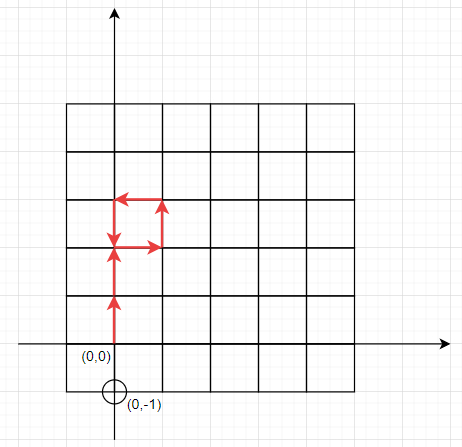# Program to check robot can reach target by keep moving on visited spots in Python

Suppose we have a robot, that is currently sitting in at position (0, 0) (Cartesian plane). If we have list of its moves that it can make, containing N(North), S(South), W(West), and E(East). However, if the robot reaches a spot it has been in before, it will continue moving in the same direction until it reaches a new unvisited spot. We have to check whether after its moves it will end at (x, y) coordinate or not.

So, if the input is likemoves = ['N','N','E','N','W','S'], coord = [0, -1], then the output will be True, as the robot will go two up, one right, one up again, one left and one down, as the current position is visited, it will go down, then that place is also visited, again down, so stop at position (0, −1)

To solve this, we will follow these steps −

• ny := 0, nx := 0

• l := a new set, initially insert coordinate (0, 0)

• for each k in moves, do

• if k is same as "N", then

• while (nx, ny) in l, do

• ny := ny + 1

• otherwise when k is same as "S", then

• while (nx, ny) in l, do

• ny := ny − 1

• otherwise when k is same as "E", then

• while (nx, ny) in l, do

• nx := nx + 1

• otherwise,

• while (nx, ny) in l, do

• nx := nx − 1

• return true when coord is same as (nx, ny), otherwise false

Let us see the following implementation to get better understanding −

## Example

Live Demo

class Solution:
def solve(self, moves, coord):
ny = nx = 0
l = {(0, 0)}
for k in moves:
if k == "N":
while (nx, ny) in l:
ny += 1
elif k == "S":
while (nx, ny) in l:
ny -= 1
elif k == "E":
while (nx, ny) in l:
nx += 1
else:
while (nx, ny) in l:
nx -= 1
return coord == nx and coord == ny

ob = Solution()
moves = ['N','N','E','N','W','S']
coord = [0,-1]
print(ob.solve(moves, coord))

## Input

['N','N','E','N','W','S'], [0,-1]

## Output

True